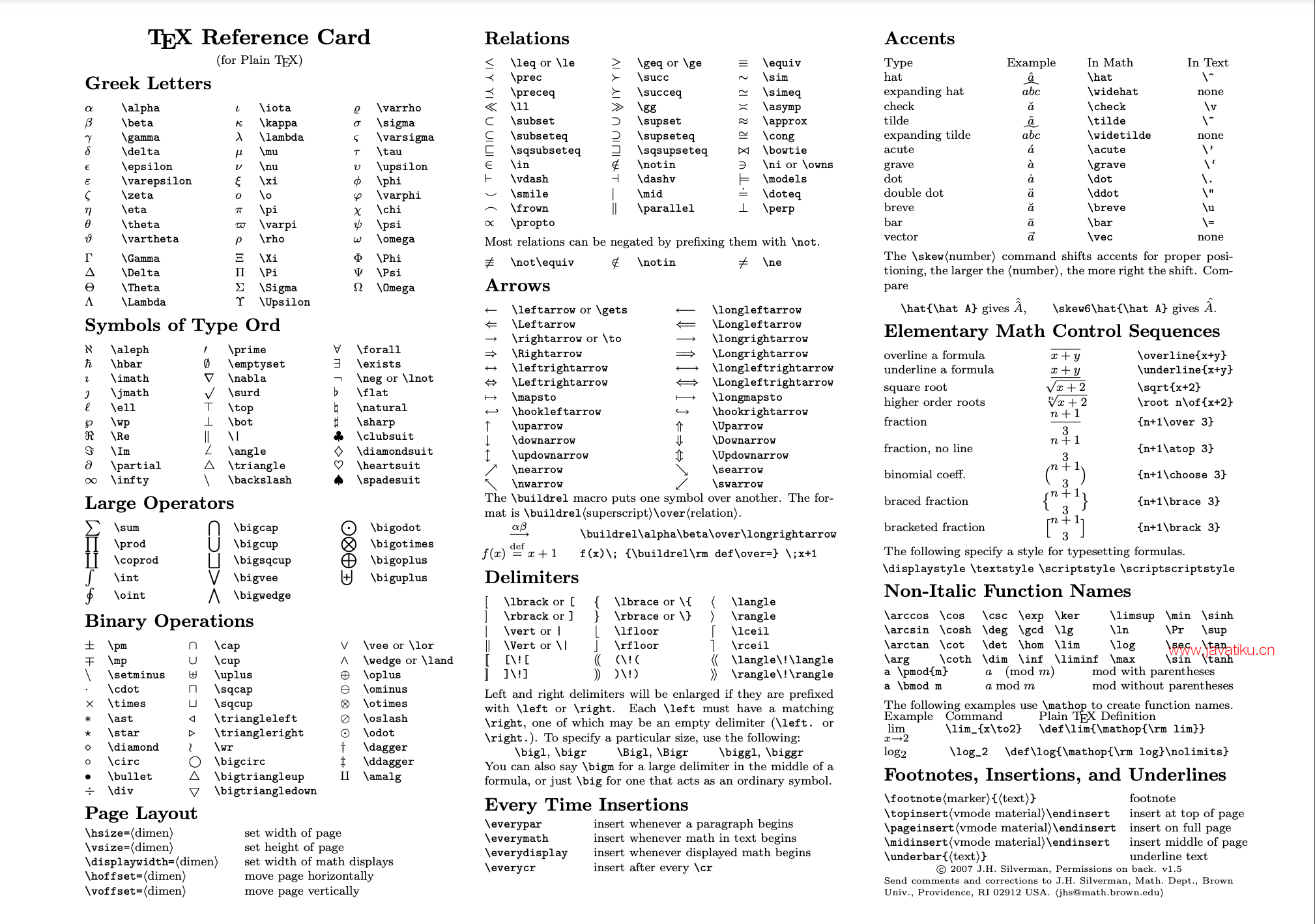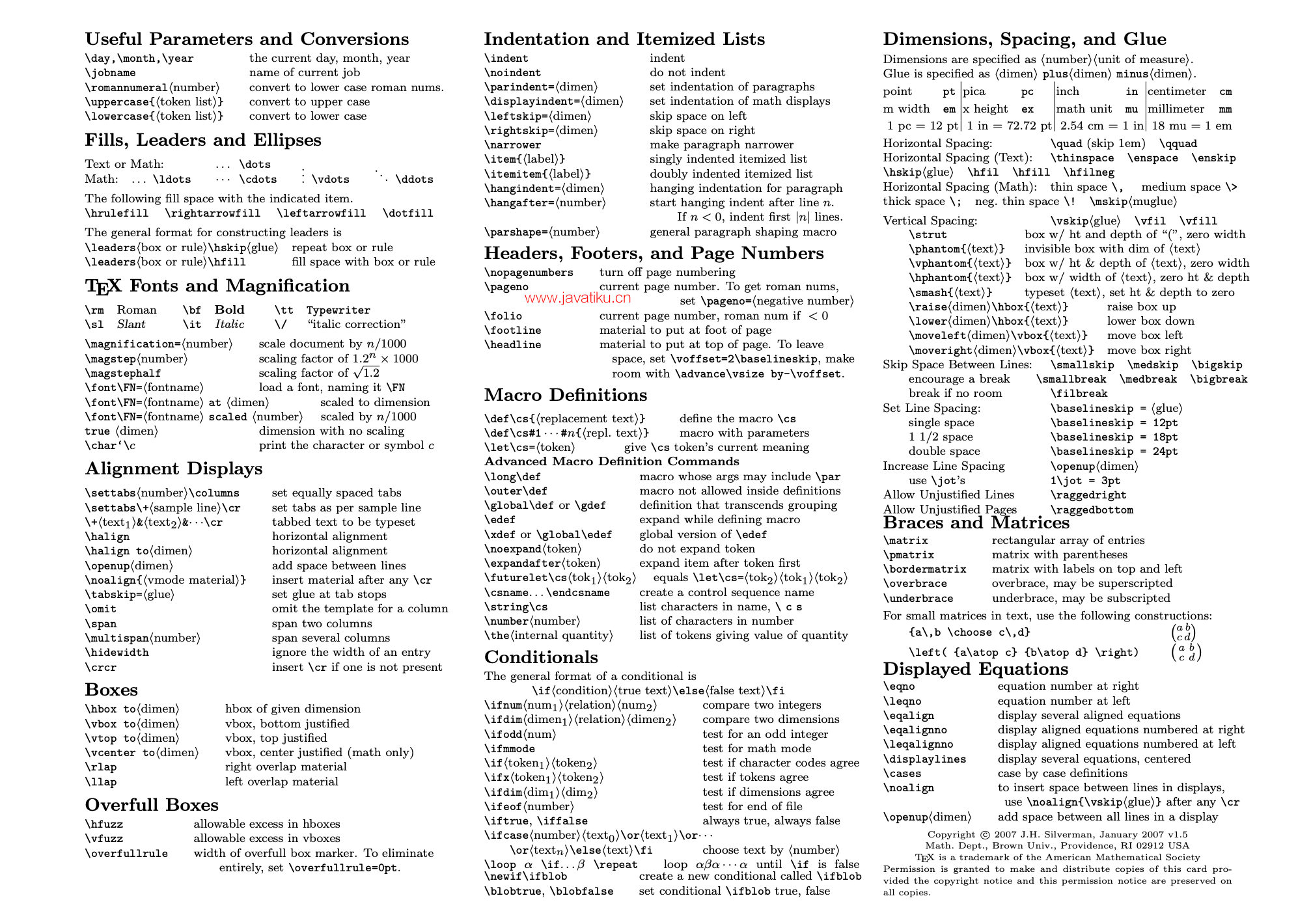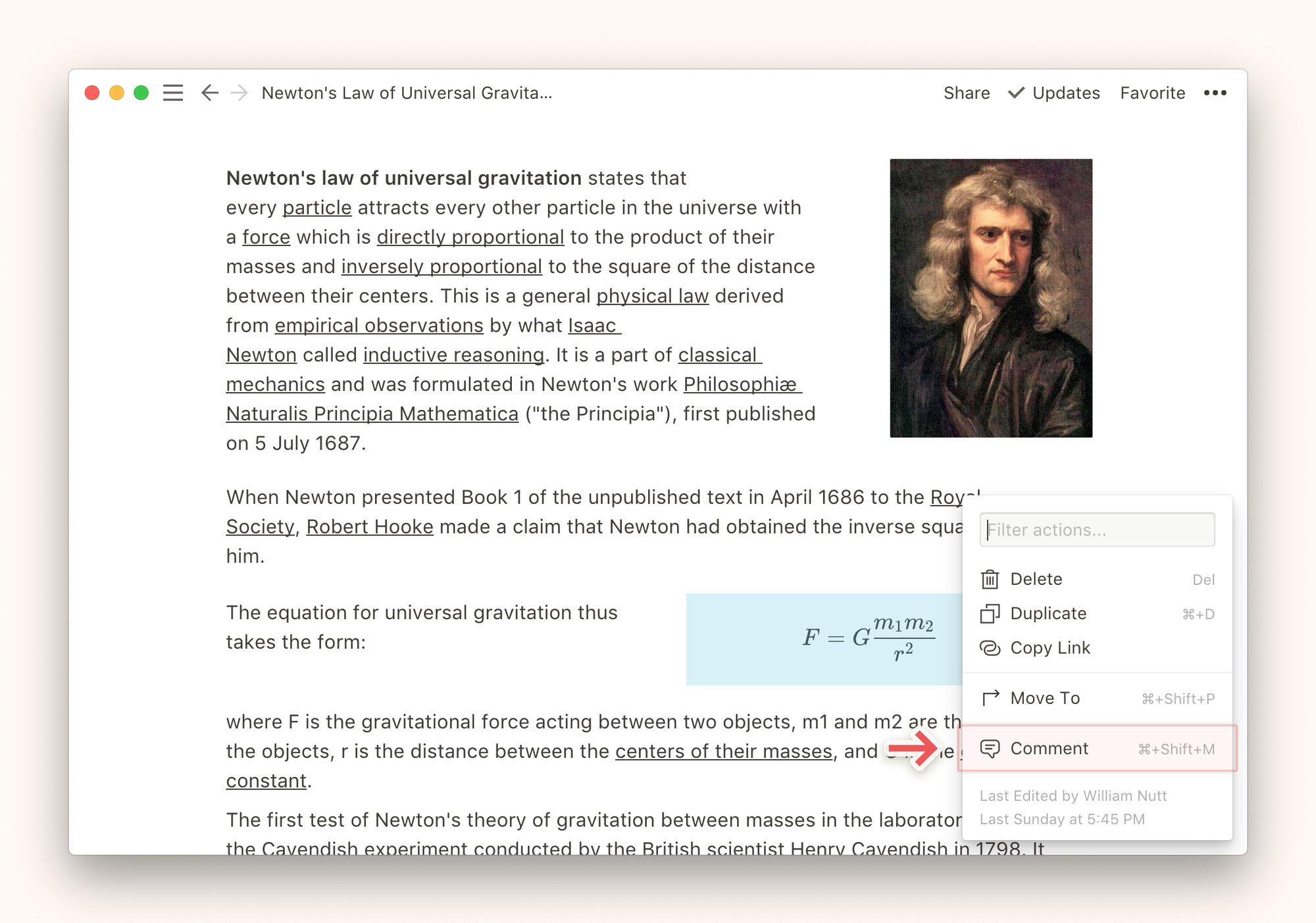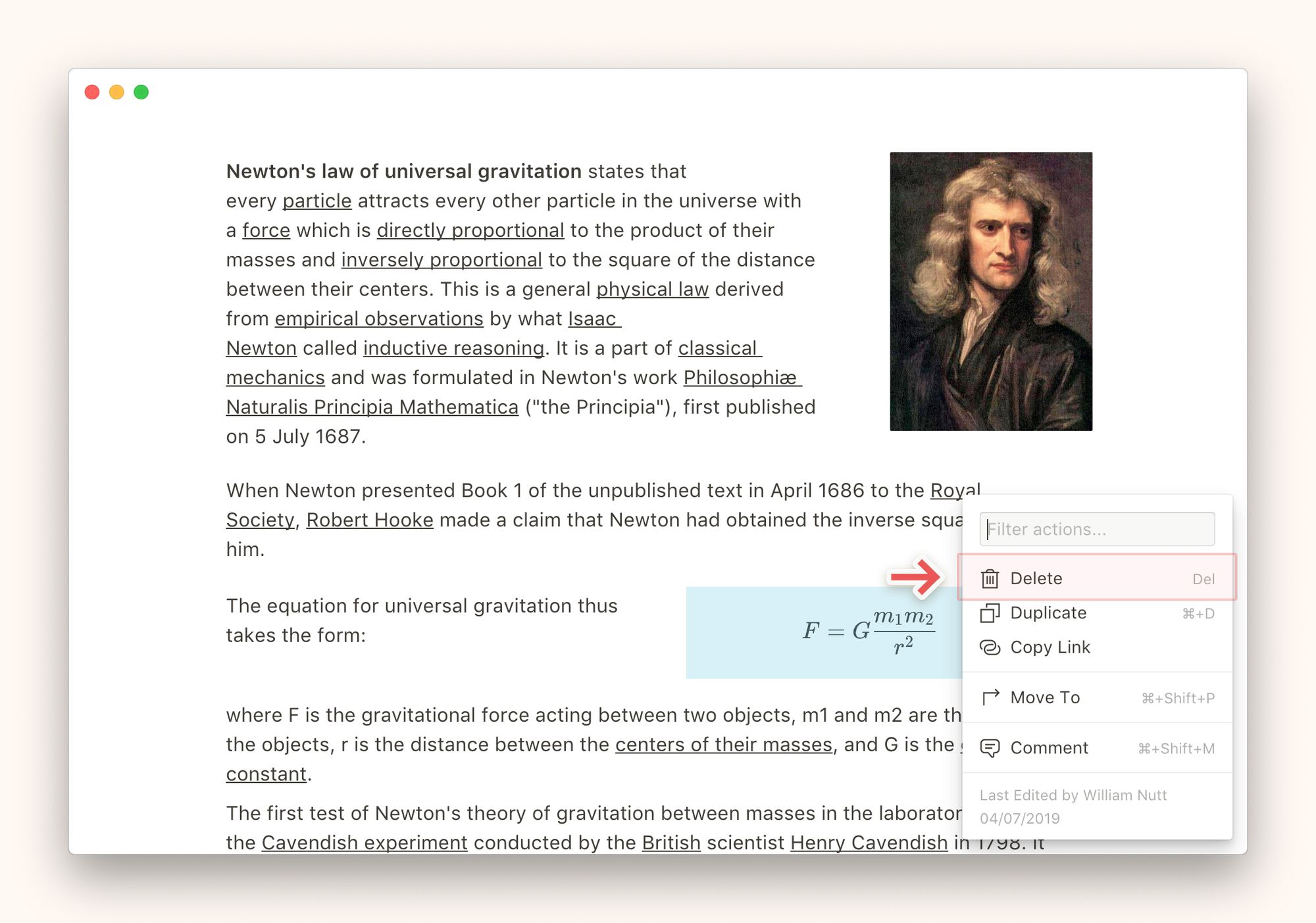# 【Notion教程】在Notion中创建数学公式## 添加math equation（数学公式）

• 当你的鼠标悬浮在一个新行上时，点击行左侧出现的 `+` 。向下滑动菜单，选择 `Math Equation（数学公式）`，然后按下 `enter`。或者，输入 `/math` 然后按下 `enter`
• 点击新出现的公式 block（块），然后输入或粘贴你的 TeX 公式。

## 可识别的 Tex 符号

Notion 支持 TeX 语言中所有的符号和操作。## 调整 math equation（数学公式）位置

• 使用拖放功能在 page（页面）上移动 math equation（数学公式）。将鼠标悬停在code block（代码块）上，然后按住手柄 `⋮⋮` 图标来实现拖动。
• 您也可以将code block（代码块）拖放到一行以分栏显示。

## 给 math equation（数学公式）添加评论

• 将鼠标悬停在 math equation（数学公式）上，然后点击右上角的 `•••` 或左侧的 `⋮⋮`。你也可以右键点击该 math equation（数学公式），或者使用快捷键 `cmd/ctrl` + `m`
• 选择 `Comment（评论）`，然后输入任意关于code（代码）的看法。## 删除 math equation（数学公式）

• 将鼠标悬浮在 math equation（数学公式）上，点击右侧的 `•••` 或者左侧的 `⋮⋮`。你也可以右键点击该 math equation（数学公式） 。
• 选择 `Delete（删除）`